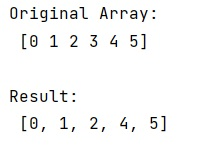# How to index every element in a list except one?

Learn, how to index every element in a list except one in Python?
Submitted by Pranit Sharma, on December 24, 2022

NumPy is an abbreviated form of Numerical Python. It is used for different types of scientific operations in python. Numpy is a vast library in python which is used for almost every kind of scientific or mathematical operation. It is itself an array which is a collection of various methods and functions for processing the arrays.

## Problem statement

Suppose we are given a NumPy array, and if we use the indexing on this, we will get the specific elements according to the positions of the elements.

We need to find a way so we will return the whole list with indexing except for a specific value.

## Indexing every element in a list except one

To index every element in a list except one, create an empty array, iterate the list elements, and insert all elements expect the given index.

The second approch that you can use - use the list comprehension to make a copy of the original array without the specific element such that we will return all the elements while indexing except that specific element.

Let us understand with the help of an example,

## Python program to index every element in a list except one

```# Import numpy
import numpy as np

# Creating a numpy array
arr = np.array([0, 1, 2, 3, 4, 5])

# Display original array
print("Original Array:\n",arr,"\n")

# Defining an empty list
res = []

# Looping over arr to make a copy
# without the specific element
for i in range(len(arr)):
if i!=3:
res.append(arr[i])
else:
continue

# Display result
print("Result:\n",res,"\n")
```

## Output

The output of the above program is: